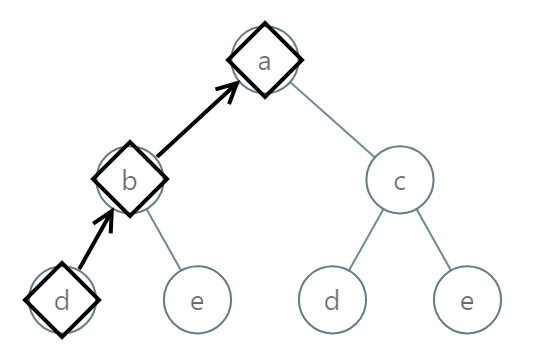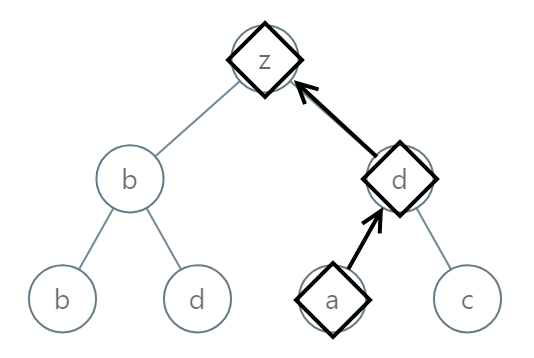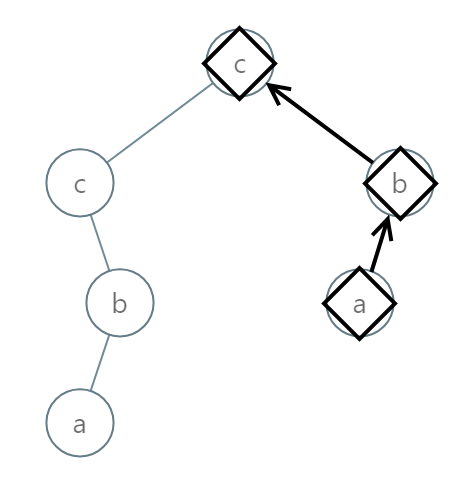# GeetCode Hub

Given the `root` of a binary tree, each node has a value from `0` to `25` representing the letters `'a'` to `'z'`: a value of `0` represents `'a'`, a value of `1` represents `'b'`, and so on.

Find the lexicographically smallest string that starts at a leaf of this tree and ends at the root.

(As a reminder, any shorter prefix of a string is lexicographically smaller: for example, `"ab"` is lexicographically smaller than `"aba"`.  A leaf of a node is a node that has no children.)

Example 1:```Input: [0,1,2,3,4,3,4]
Output: "dba"
```

Example 2:```Input: [25,1,3,1,3,0,2]
```

Example 3:```Input: [2,2,1,null,1,0,null,0]
Output: "abc"
```

Note:

1. The number of nodes in the given tree will be between `1` and `8500`.
2. Each node in the tree will have a value between `0` and `25`.

/** * Definition for a binary tree node. * public class TreeNode { * int val; * TreeNode left; * TreeNode right; * TreeNode() {} * TreeNode(int val) { this.val = val; } * TreeNode(int val, TreeNode left, TreeNode right) { * this.val = val; * this.left = left; * this.right = right; * } * } */ class Solution { public String smallestFromLeaf(TreeNode root) { } }